# Dedekind-Hasse norm implies principal ideal ring

(diff) ← Older revision | Latest revision (diff) | Newer revision → (diff)

## Statement

A commutative unital ring that admits a Dedekind-Hasse norm must be a principal ideal ring.

In particular, an integral domain that admits a Dedekind-Hasse norm must be a principal ideal domain.

## Definitions used

### Dedekind-Hasse norm

Further information: Dedekind-Hasse norm

A Dedekind-Hasse norm on a commutative unital ring$R$ is a function$N$ from the nonzero elements of$R$ to the nonnegative integers with the property that for any elements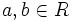$a,b \in R$ with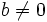$b \ne 0$, it is true that either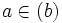$a \in (b)$ or there exists an element$r$ in the ideal$(a,b)$ such that$N(r) < N(b)$.

### Principal ideal ring

Further information: Principal ideal ring

A commutative unital ring$R$ is termed a principal ideal ring if every ideal of$R$ is principal.

## Proof

Given: A commutative unital ring$R$ with a Dedekind-Hasse norm$N$. An ideal$I$ of$R$.

To prove: There exists$b \in I$ such that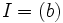$I = (b)$.

Proof: If$I = 0$, we can take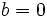$b = 0$, and we are done.

So, suppose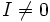$I \ne 0$. Consider the function$N$ on the nonzero elements of$I$. Since$N$ maps to a well-ordered set, there is an element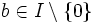$b \in I \setminus \{ 0 \}$ such that$N(b) \le N(r)$ for all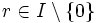$r \in I \setminus \{ 0 \}$.

Pick any$a \in I$. If$a = 0$, then$a \in (b)$ or there exists$r \in (a,b)$ with$N(r) < N(b)$.

Note that in the latter case, we have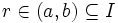$r \in (a,b) \subseteq I$, so$r \in I$, and$N(r) < N(b)$, contradicting the assumption that$b$ has minimum norm among the nonzero elements of$I$. Hence, we have the case$a \in (b)$. Thus,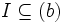$I \subseteq (b)$. Conversely, we clearly have$(b) \subseteq I$, so$I = (b)$.